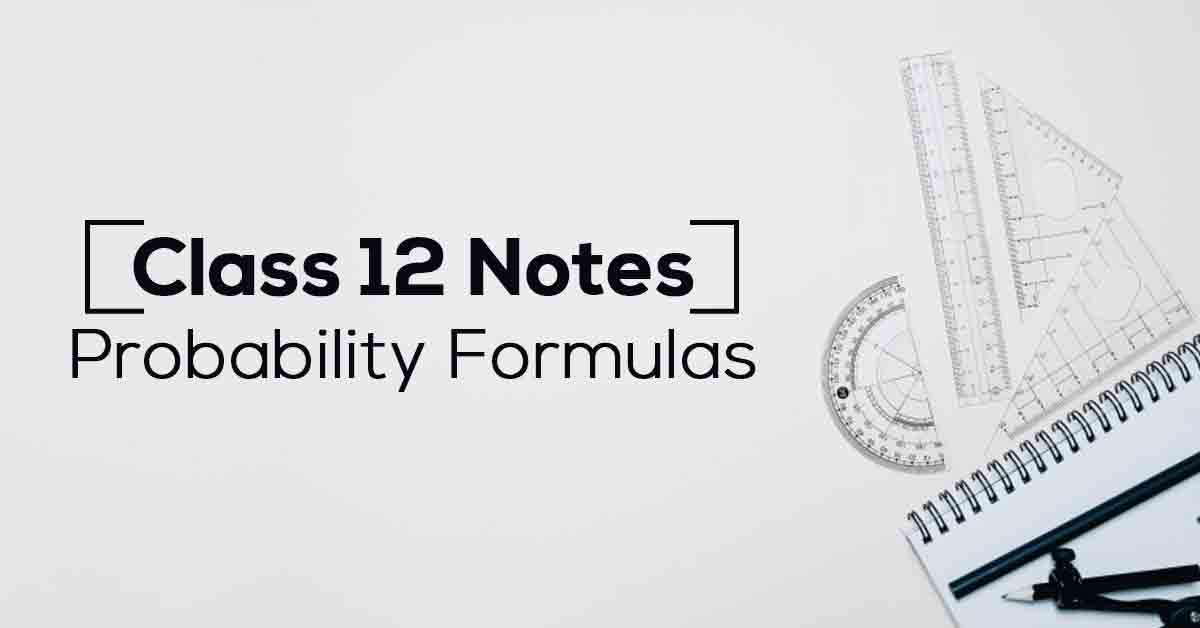Probability Class 12 Formulas PDF with Notes | Vidyakul
×

# Probability Class 12 Formulas PDF with NotesProbability is the measure of the likelihood that an event will occur. Probability is quantified as a number between 0 and 1, where, loosely speaking, 0 indicates impossibility and 1 indicates certainty. Know More about these in Probability Class 12 Formulas PDF with Notes List.

The topics and sub-topics covered in Probability Class 12 Formulas PDF with Notes are:

13.1 Introduction

13.2 Conditional Probability

13.2.1 Properties of Conditional Probability

13.3 Multiplication Theorem on Probability

13.4 Independent Events

13.5 Baye’s Theorem

13.5.1 Partition of a Sample Space

13.5.2 Theorem of Total Probability

13.6 Random Variables and its Probability Distributions

13.6.1 Probability Distribution of a Random Variable

13.6.2 Mean of a Random Variable

13.6.3 Variance of a Random Variable

13.7 Bernoulli’s Trials and Binomial Distribution

13.7.1 Bernoulli Trials

13.7.2 Binomial Distribution.

Download the FREE PDF of Probability Class 12 Formulas PDF with Notes and start your preparation with Vidyakul!